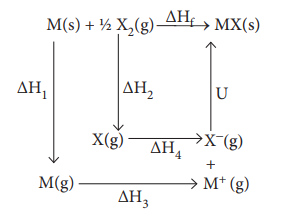Home | | Chemistry 11th std | Born - Haber cycle for determining the lattice enthalpy of NaCl

# Born - Haber cycle for determining the lattice enthalpy of NaCl

The BornŌĆōHaber cycle is an approach to analyse reaction energies.

Born - Haber cycle

The BornŌĆōHaber cycle is an approach to analyse reaction energies. It was named after two German scientists Max Born and Fritz Haber who developed this cycle. The cycle is concerned with the formation of an ionic compound from the reaction of a metal with a halogen or other non-metallic element such as oxygen.

BornŌĆōHaber cycle is primarily used in calculating lattice energy, which cannot be measured directly. The BornŌĆōHaber cycle applies Hess's law to calculate the lattice enthalpy. For example consider the formation of a simple ionic solid such as an alkali metal halide MX, the following steps are considered.ŌłåH1 - enthalpy change for the sublimation M(s) to M(g)

╬öH2- enthalpy change for the dissociation of ┬Į X2 (g) to X(g)

ŌłåH3- Ionisation energy for M(g) to M+(g) ŌłåH4 - electron affinity for the conversion of X(g) to X-(g)

U - the lattice enthalpy for the formation of solid MX

ŌłåHf - enthalpy change for the formation of solid MX directly form elements According to Hess's law of heat summation

ŌłåHf  =  ŌłåH1  +  ŌłåH 2 +  ŌłåH 3 +  ŌłåH 4 + U

Let us use the Born - Haber cycle for determining the lattice enthalpy of NaCl as follows:

Since the reaction is carried out with reactants in elemental forms and products in their standard states, at 1 bar, the overall enthalpy change of the reaction is also the enthalpy of formation for NaCl. Also, the formation of NaCl can be considered in 5 steps. The sum of the enthalpy changes of these steps is equal to the enthalpy change for the overall reaction from which the lattice enthalpy of NaCl is calculated.

Let us calculate the lattice energy of sodium chloride using Born-Haber cycle╬öHf = heat of formation of sodium chloride = ŌĆō 411.3 kJ molŌĆō1

╬öH1 = heat of sublimation of Na(S) = 108.7 kJ molŌĆō1

╬öH2 = ionisation energy of Na(S) = 495.0 kJ molŌĆō1

╬öH3 = dissociation energy of Cl2(S) = 244 kJ molŌĆō1

╬öH4 = Electron affinity of Cl(S)= ŌĆō 349.0 kJ molŌĆō1

= lattice energy of NaCl

ŌłåHf = ŌłåH+ ŌłåH+ 1/2ŌłåH+ ŌłåH+U

U = (ŌĆō411.3) ŌĆō (108.7 + 495.0 + 122 ŌĆō 349)

U = (ŌĆō411.3) ŌĆō (376.7)

U = ŌĆō 788 kJ molŌĆō1

This negative sign in lattice energy indicates that the energy is released when sodium is formed from its constituent gaseous ions Na+ and Cl-

Study Material, Lecturing Notes, Assignment, Reference, Wiki description explanation, brief detail
11th Chemistry : UNIT 7 : Thermodynamics : Born - Haber cycle for determining the lattice enthalpy of NaCl |